# Deciding How Much Bandwidth Is Enough

Once you understand how to calculate the bandwidth needed to support a certain number of voice calls, using a certain CODEC with certain options (for example, payload size, media type, and any extra overhead for security), the next step is to determine how much bandwidth is needed to support the busiest telephone usage time of the day for a company. However, before you can determine the required bandwidth, you first need to know how many calls you need to support simultaneously. Then you can simply feed that number of calls into the Cisco web-based Voice Bandwidth Calculator discussed earlier.

You must first figure out how many telephone calls are going on during the busiest time of the day for your telephone network. If we dug into all of the nuts and bolts of doing this calculation, it could get very complicated. Voice professionals even have a name for this type of calculation: traffic engineering.

Traffic engineering gets deep into statistical analysis and very complex math. However, in this chapter, you're going to learn some great shortcuts, and these shortcuts provide excellent results, without having to dust off the scientific calculator you had in college. Here is the game plan:

 Step 1. Calculate Erlangs based on monthly private branch exchange (PBX) usage. Step 2. Determine the grade of service (GoS) (that is, how many rejected calls are acceptable during the busiest hour of the day). Step 3. Calculate the number of required trunks, based on the Erlang value and the GoS value, using a web-based Erlang B calculator. Step 4. Calculate the amount of required bandwidth, using the Cisco web-based Voice Bandwidth Calculator.

The following sections walk through each step.

#### Step 1: Calculating Erlangs

The call volume experienced by a company's telephone system (for example, PBX) is measured in Erlangs, where an Erlang is one solid hour of phone usage. For example, if you and three of your coworkers each use your company's phone system for 30 minutes during the same hour, that's a total of 120 call minutes (that is, 4 * 30) of phone system use. Because an Erlang is measured in hours, you can divide the call minutes by 60 to convert the call minutes to Erlangs. In this example, you and your coworkers had 2 Erlangs worth of phone system usage (that is, 120 / 60).

However, our traffic engineering goal for Step 1 is to determine the number of Erlangs of phone system usage your company experiences during the busiest hour of the day. There are various approaches to calculating the busy hour traffic, but let's consider an approach that only requires you to know the total number of minutes your company's phone system used during an entire month. This "call minutes" value could come from your company's internal Station Message Detail Recorder (SMDR) information or from your company's phone bill.

Statistically, the number of call minutes a corporate phone system experiences during the busiest hour of the day can be approximated using the following formula:

Busy_Hour_Call_Minutes = [Monthly_Call_Minutes / 22] * .15

The rationale for this formula is based on the observation that a month contains approximately 22 business days, and during a business day, approximately 15 percent of a day's call volume occurs during the busiest hour of the day. We can then calculate the busy hour Erlang value by dividing the Busy Hour Call Minutes by 60.

As an example, consider a scenario where a company's phone bill shows 50,000 minutes of phone usage during a month. We can calculate the company's busy hour call minutes as follows:

Busy_Hour_Call_Minutes = [50,000 / 22] * .15 = 340.9 call minutes

Next, we can convert the call minutes value into Erlangs by dividing the call minutes value by 60:

Erlangs = 341 / 60 = 5.7

#### Step 2: Determining the Grade of Service

Now that we know the call volume during your company's busy hour, we make the observation that we probably don't want to accommodate every single call during that busy hour. After all, if we purchased sufficient trunks (in the PBX world) or sufficient bandwidth (in the VoIP world) to handle every call during the busy hour, some trunks, or bandwidth, would go unused during the remainder of the day. Therefore, we need to determine an acceptable percentage of calls to reject during the busiest hour of the day. This percentage is defined as a grade of service (GoS). Typically, telephone network designers use a GoS of P(.01), which is a one percent chance that a call will be rejected during the busiest hour of the day.

#### Step 3: Calculating the Number of Required Trunks

After calculating the call volume we need to support (measured in Erlangs) and determining the percentage of calls we're willing to reject during the busiest hour of the day (specified as a GoS), we can use an Erlang B calculator to determine how many trunks are required for a PBX to handle this call volume.

note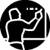Erlang B is not the only Erlang model. Other models can be used for applications such as sizing a call center. However, for a company's telephone system, the Erlang B model is usually appropriate.

The web-based Erlang B calculator located at http://erlang.com/calculator/erlb takes our Erlang and GoS values and calculates the required number of trunks to accommodate the busy hour traffic with a specific GoS. Consider our example, with 5.7 Erlangs and a GoS of P(.01). We enter the 5.7 value in the BHT (Erl.) field of the Erlang B calculator (where BHT represents Busy Hour Traffic measured in Erlangs) and .01 in the Blocking field (which is the default value), as shown in Figure 2-11.

##### Figure 2-11. Erlang B Calculator Inputting Busy Hour Traffic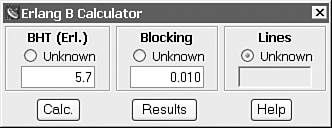We then click the Calc. button, and the calculator displays the number of trunks needed to support the specified call volume, as shown in Figure 2-12. In our example, we require 12 trunks.

##### Figure 2-12. Erlang B Calculator Calculating Lines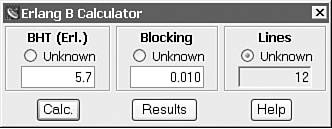#### Step 4: Calculating the Amount of Required Bandwidth

PBX traffic engineers would be done at this point. They would know how many trunks the PBX needed. However, we need to take it a step further and determine how much bandwidth is required to support the specified call volume, and the good news is that we already know how to do it. Do you remember when we used the Cisco web-based Voice Bandwidth Calculator? We can use that same calculator again, inserting the number of required trunks (that is, 12 trunks), as calculated in Step 3, in the Number of Calls field, as shown in Figure 2-13.

##### Figure 2-13. Calculating the Required Bandwidth for 12 Trunks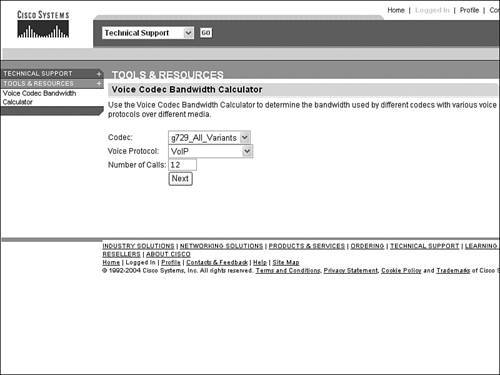When sending these calls across the IP WAN, let us assume the following:

• CODEC: G.729a

• Voice Protocol: VoIP

• Voice Payload Size: 20 Bytes (default)

• RTP Header Compression: Disabled

• Media Access: PPP

• Tunnel/Security/Misc. Overhead: None

After entering these parameters into the Cisco Voice Bandwidth Calculator, we get a result of 337.68 kbps of bandwidth required to support the call volume in our example.

Admittedly, we went through this traffic engineering calculation slowly to demonstrate the details of each step. However, in the real world you can calculate the required voice bandwidth very rapidly. Remember the four steps:

 Step 1. Determine your company's call minutes from your phone bill, divide by 22, and multiply by .15. Divide that number by 60 to calculate your Erlang requirements. Step 2. Typically, you will choose a GoS of P(.01). Step 3. Enter your Erlang and GoS values into the web-based Erlang B calculator to determine the number of required trunks. Step 4. Enter the number of trunks and your specific VoIP call parameters in the Cisco Voice Bandwidth Calculator to determine the required voice bandwidth.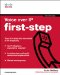Voice over IP First-Step
ISBN: 1587201569
EAN: 2147483647
Year: 2005
Pages: 138
Authors: Kevin Wallace

Similar book on Amazon

flylib.com © 2008-2017.
If you may any questions please contact us: flylib@qtcs.net